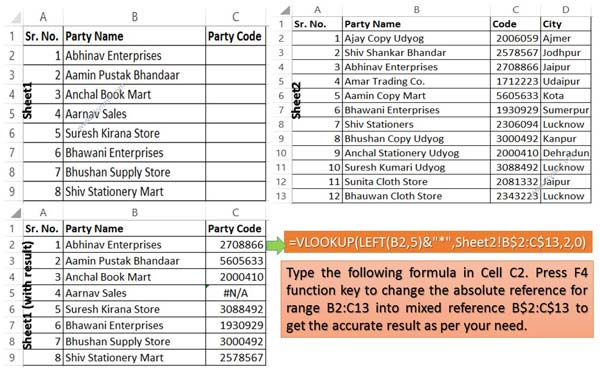# VLookup to find first few characters in Excel

VLookup to find first few characters in Excel article helps you to search first few characters of any name to get the proper result in Excel.

All we know VLookup function play an important role in MS Excel. It is used to search any specific value in the selected range of cells. Sometime we need to lookup few characters of one column to another. This guide helps you how to use VLookup to find first few characters in Excel.

“VLookup” is one of the most important lookup functions which is located in the “Lookup and Reference” category of Microsoft Excel.

For e.g.: If you have an large database in which you have to fill party code using VLookup function in Microsoft Excel. First worksheet have three columns “Sr. No.”, “Party Name” & “Code” while second worksheet have four columns “Sr. No.”, “Party Name”, “Code” & “City”. Now if you want to fill code using VLookup to find first few characters in Excel.

Before using the VLookup function first we have to know the brief description of VLookup in Microsoft Excel.

### Syntax of VLookup function:

The Microsoft Excel VLookup function is used to search specific value in the extreme left column of the table_array. It provides the value in the same row which is based on the index number.

= VLookup (lookup_value, table_array, col_index_num, [range])

Note: VLookup function is used to search the value of extreme left column. So, you have to arrange first column of any table or array in ascending order, otherwise VLookup function is not able to provide the accurate result.

Lookup_value: The value to be searched in the extreme first column of the table array.

Table_array: Two or more columns of data. Give cell reference of a range / range name.

Col_index_num: The column index_number in the table from which the searched value must be returned.

If col_index_num of returns 1 the value in the first column in table_array.

If col_index_num is: Less than 1, this function returns an error value the #VALUE!.

If it is a greater than the number of columns in table_array, this function returns error value #REF!.

Range_lookup: It is an options part of this function. There are two options available in this part.

TRUE: Appropriate Match: This option work properly when first column of your table will be sorted in ascending order only otherwise it displays wrong
result.

False: Exact Match: VLookup by default check only on exact match method.

## VLookup to find first few characters in Excel

If you have a large worksheet data and want to search specific value which is based on the first five characters. Now, you have to take following few simple steps for VLookup to find first few characters in Excel.Step 1: Create following given two worksheets in your active workbook.

Step 2: If you want to search and fill party code in “C” column of Sheet1 then you have to type the following given formula in cell C2:

=VLOOKUP(LEFT(B2,5)&”*”,Sheet2!B2:C13,2,0)

Step 3: Again double click on cell C2 and change the absolute reference type of range B2:C13 in mixed reference B\$2:C\$13. You have to select the range B2:C13 and press F4 function key to change the reference type. Now, your formula is shown like this in cell C2:

=VLOOKUP(LEFT(B2,5)&”*”,Sheet2!B\$2:C\$13,2,0)

Step 4: If you don’t want to type the formula for rest of the cells than drag the fill handle of cell C2 to C9. It will automatically copy the formula and calculate the result for rest of the cells.

I hope this VLookup to find first few characters in Excel article is helpful for all Excel users. This guide is useful for those guys who are facing the problem during the searching the name which is based on few characters. If you have any suggestion or query then write us in the comment box. Thanks to all.